Mathieu functions

(diff) ← Older revision | Latest revision (diff) | Newer revision → (diff)

The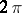-periodic solutions of the Mathieu equation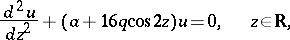which exist only when the point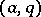in the parameter plane lies on the boundary of the stability zones. A Mathieu function is even or odd, and is unique up to a factor; the second linearly-independent solution grows linearly infor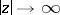, provided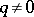. The even Mathieu functions are the eigen functions of the integral equation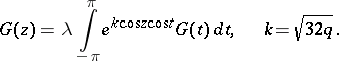An analogous equation is satisfied by the odd Mathieu functions. The notation for Mathieu functions is: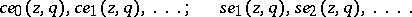For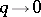these functions reduce to the trigonometric systemand they possess the same orthogonality properties on the interval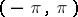. The Mathieu functions admit Fourier-series expansions which converge for small; the coefficients of these series are convergent power series in, for example,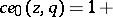How to Cite This Entry:
Mathieu functions. Encyclopedia of Mathematics. URL: http://encyclopediaofmath.org/index.php?title=Mathieu_functions&oldid=14316
This article was adapted from an original article by M.V. Fedoryuk (originator), which appeared in Encyclopedia of Mathematics - ISBN 1402006098. See original article